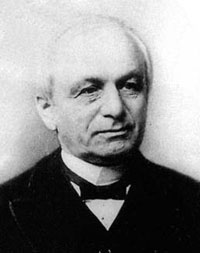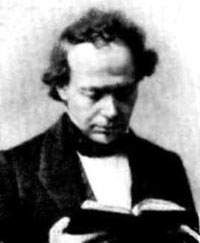On page 227 of Symmetry and the Monster, Mark Ronan tells the story of Conway and Norton computing the number of independent _mini j-functions_ (McKay-Thompson series) arising from the Moonshine module. There are 194 distinct characters of the monster (btw. see the background picture for the first page of the character table as given in the Atlas), but some of them give the same series reducing the number of series to 171. But, these are not all linearly independent. Mark Ronan writes :

“Conway recalls that, ‘As we went down into the 160s, I said let’s guess what number we will reach.’ They guessed it would be 163 – which has a very special property in number theory – and it was!
There is no explanation for this. We don’t know whether it is merely a coincidence, or something more. The special property of 163 in number theory has intruiging consequences, among which is the fact that
$e^{\pi \sqrt{163}} = 262537412640768743.99999999999925…$
is very close to being a whole number.”

The corresponding footnote is a bit cryptic and doesn’t explain this near miss integer.

“This special feature also yields a fact, first noticed by Euler, that the formula
$x^2-x+41$
gives prime numbers for all values of x between 1 and 40. The connection with 163 is that the solution to
$x^2-x+41=0$
involves the square root of -163.”

So, what is really going on?

The _modular j-function_ has a power series expansion in $q=e^{2 \pi i \tau}$ starting off as

$j(\tau) = q^{-1} + 744 + 196884 q + 21493760 q^2 + 864299970 q^3 + \cdots$

and classifies complex elliptic curves upto isomorphism, or equivalently, two-dimensional integral lattices upto a complex scaling factor. A source of two-dimensional integral lattices is given by the rings of integers $\mathbb{Z}.1 + \mathbb{Z}.\tau$ in quadratic imaginary extensions of the rational numbers $\mathbb{Q}(\sqrt{-D})$. So, perhaps one might expect special properties of the j-value $j(\tau)$ whenever this ring of integers has special properties.Leopold Kronecker discovered in 1857 the remarkable fact that the modular j-function detects the class number of $\mathbb{Q}(\sqrt{-D})$. Recall that the class-number is a finite number measuring the amount by which the ring on integers $\mathbb{Z}.1 + \mathbb{Z}.\tau$ in $\mathbb{Q}(\sqrt{-D})$ fails to be a unique factorization domain. He proved :

The function-value $j(\tau)$ is an algebraic integer whose degree is the class number of the quadratic extension. In particular, if the ring of integers in $\mathbb{Q}(\sqrt{-D})$ satisfied unique factorization (or equivalently, is a principal ideal domain), then $j(\tau)$ is an integer!

Special instances of this theorem were already known. For example, the Gaussian integers $\mathbb{Z}.1+\mathbb{Z}.i$ satisfy unique factorization and Gauss knew already that $j(i)=12^3=1728$. He even knew that

$j(\frac{1}{2}(1+\sqrt{-163}))=(-640320)^3=-262537412640768000$

and because $\mathbb{Z}.1+\mathbb{Z}.\frac{1}{2}(1+\sqrt{-163})$ is the ring of integers in $\mathbb{Q}(\sqrt{-163})$ and as the absolute value of this j-value is near the value of $e^{\pi \sqrt{163}}$ we must be closing in on the solution of the riddle.Charles Hermite noticed in 1859 this curious numerical consequence of Kronecker’s theorem. For, if one takes $\tau = \frac{1}{2}(1+\sqrt{-163})$ and plugs this into the definition of $q=e^{2 \pi i}$ one gets the tiny number
$q = e^{\pi i – \pi \sqrt{163}} = – e^{-\pi \sqrt{163}}$ which is equal to -0.0000000000000000038089809370076523382…
So, all but the first two terms in the series expansion of $j(\tau)$ will be very small. For example
$196884 q = -0.00000000000074992740…$ and the next term $21493760 q^2$ is already 0.00000000000000000000000000031183868… and further terms will be even a lot smaller.

Combining this information with the Gauss-computed value of $j(\tau)$ we get that

$~(-640320)^3 = -e^{\pi \sqrt{163}} + 744 – \text{tiny number}$

whence the observed curious approaximation of

$e^{\pi \sqrt{163}} = 262537412640768000 + 744 -\text{tiny number} = 262537412640768743.99999999999925…$

What about other near misses which follow from Kronecker’s result? Unfortunately there are only nine imaginary quadratic extension $\mathbb{Q}(\sqrt{-D})$ for which the corresponding ring of integers satisfies unique factorization, namely

$D=1,2,3,7,11,19,43,67,163$

and of course the near misses will be worse for smaller values of D. For example for the next two largest values one calculates

$e^{\pi \sqrt{67}} = 147197952743.99999866245422…$

$e^{\pi \sqrt{43}} = 884736743.99977746603490661…$

Reference :

John Stillwell, Modular Miracles, The American Mathematical Monthly, 108 (2001) 70-76

Published in stories